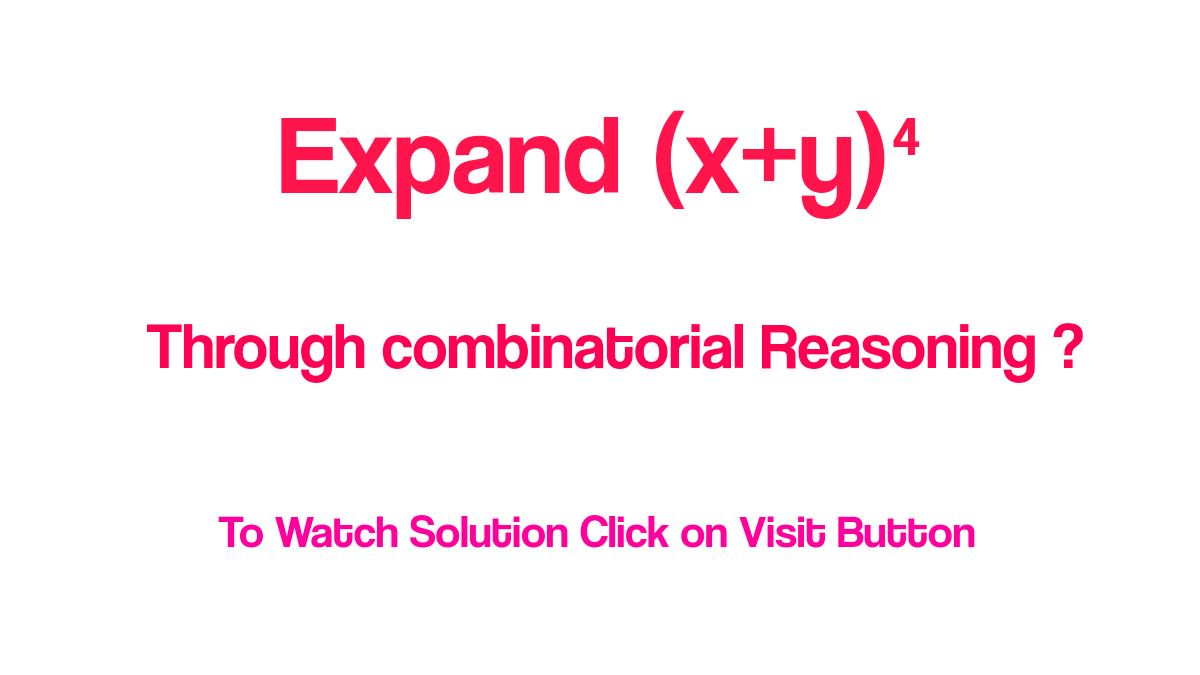# Expand (x+y)⁴ through combinatorial reasoning

Here we use combinatorial reasoning to expand (x+y)⁴ instead of multiplication of (x+y) four times.Expand (x+y)⁴ through combinatorial reasoning

So first of all expand (x+y)⁴ as given below

(X+y)⁴ = (x+y)(x+y)(x+y)(x+y)

Number of sum=n=4

Following terms are arises X⁴,Y⁴,XY³,YX³,X²Y² when combinatorial reasoning is applied same as given below;

1-Pick X from a first sum, second sum,third sum and fourth sum and then multiply same as (X)(X)(X)(X)(X) = X⁴ . This is done in one way because X⁴ has only 1 as a coefficient that is obtained by taking n as total number of sums and r as power of X thus C(4 , 4 ) = 1

2-Now a pick X from first sum, Y from second Sum,Y from third sum and Y from fourth sum and then multiply as

XYYY=XY³

This can be done in 4 ways means XY³ has 4 as coefficient obtained from C(4 , 1 ) = 4 taking X power as r and total number of sum as n.

So there is 4XY³

3-Now a pick Y from first sum, X from second Sum,X from third sum and X from fourth sum and then multiply as

YXXX=YX³

This can be done in 4 ways means YX³ has 4 as coefficient obtained from C(4 , 1 ) = 4 Here Y power is used as r and total number of sum as n

So there is 4XY³

4-Now a pick Y from first sum, Y from second Sum,Y from third sum and Y from fourth sum and then multiply as

YYYY=Y⁴

This can be done in 1 ways means Y⁴ has 1 as coefficient obtained from C(4 , 4 ) = 4 By using power of Y as r and total number of sum as n

So there is Y⁴

5- Pick X from first Sum ,X from second Sum,Y from third sum,Y from fourth sum and then multiply as

XXYY = X²Y² This can be done in 6 ways because it has 6 as coefficient obtained from C(4 , 2 ) = 6 taking power of X or Y as r and number of sum as n.

So there is 6X²Y²

#### 6-Now add all products that is the expansion of (x+y)⁴(x+y)⁴ = X⁴ + 4X³Y + 4XY³ + 6X²Y² + Y⁴

Hence we have been expand (X+Y)⁴ by combinatorial reasoning.